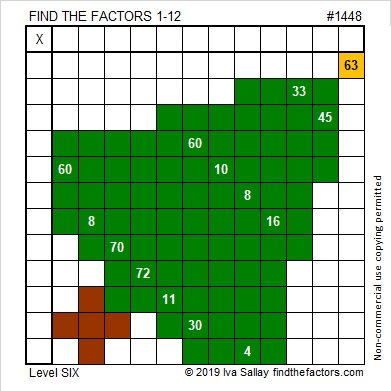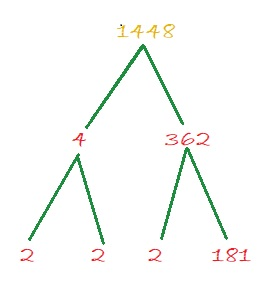# 1448 Christmas Factor Tree

Here’s a puzzle that looks a little like a Christmas tree. Some of the clues might give you a little bit of trouble. For example, the common factor of 60 and 30 might be 5, 6, or 10. Likewise, the common factor of 8 and 4 might be 1, 2, or 4.

Which factor should you use? Look at all the other clues and use logic. Logic can help you write each of the numbers 1 to 12 in both the first column and the top row so that the given clues and those numbers behave like a multiplication table. Good luck!I have to number every puzzle. It won’t help you solve the puzzle, but here are some facts about the number 1448:

The number made by its last two digits, 48, is divisible by 4, so 1448 is also divisible by 4. That fact can give us the first couple of branches of 1448’s factor tree:• 1448 is a composite number.
• Prime factorization: 1448 = 2 × 2 × 2 × 181, which can be written 1448 = 2³ × 181
• 1448 has at least one exponent greater than 1 in its prime factorization so √1448 can be simplified. Taking the factor pair from the factor pair table below with the largest square number factor, we get √1448 = (√4)(√362) = 2√362
• The exponents in the prime factorization are 3 and 1. Adding one to each exponent and multiplying we get (3 + 1)(1 + 1) = 4 × 2 = 8. Therefore 1448 has exactly 8 factors.
• The factors of 1448 are outlined with their factor pair partners in the graphic below.1448 is also the hypotenuse of a Pythagorean triple:
152-1440-1448 which is 8 times (19-180-181)

This site uses Akismet to reduce spam. Learn how your comment data is processed.